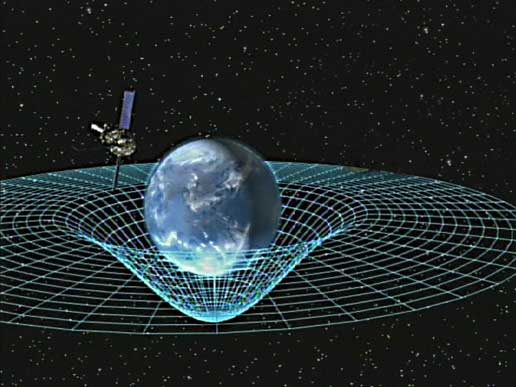# Specific Solution in GR

benk99nenm312
I have a question on finding a specific solution to Einstein's Field Equations. This is purely for my own curiosity. Suppose you were given this picture.How would you find the curvature of the point directly beneath the Earth in the picture, i.e. the point in which the space-time is dipped down the most? It should be the highest curvature in local space. What type of solution would this be?

If this doesn't make sense, please tell me so that I can re-word it in a way that does.

How would you find the curvature of the point directly beneath the Earth in the picture,
I guess you mean "in the center of the Earth". The picture is a bit misleading
i.e. the point in which the space-time is dipped down the most?
It is important to state, that the picture shows purely spatial curvature. There is no time dimension represented in that diagram.

It should be the highest curvature in local space.
Not really. The local space curvature itself could be even constant in the entire interior of a mass sphere. What you mean is the lowest gravitational potential.

What type of solution would this be?

http://de.wikipedia.org/wiki/Schwarzschild-Metrik#Geometrische_Deutung_2" says, that the spatial part of the metric represents a Hypersphere, which would suggest an uniform space curvature in the entire interior.

Last edited by a moderator:
benk99nenm312
Interesting. So the answer doesn't even lie in EFE? It's Schwarchild's equation?

So, in what units is this measured in? (I'm extremely new to the math of Relativity)

Mentz114
That diagram and all it's simulacra are misleading. It's much better to think of the spatial curvature around the earth as a series of concentric spherical shells getting further apart as they get further from the surface. That will give an idea of the magnitude of the spatial curvature, but as A.T. has said, in GR the curvature is in 4 dimensions.

Interesting. So the answer doesn't even lie in EFE? It's Schwarchild's equation?
The Schwarchild metric is a solution of the EFE. Your picture visualizes the spatial part of the Schwarchild metric. In fact it shows only 2 of the 3 space dimensions and 4 spacetime dimension.
So, in what units is this measured in? (I'm extremely new to the math of Relativity)
Usually http://en.wikipedia.org/wiki/Geometrized_unit_system" [Broken] is quite complex.

Last edited by a moderator:
benk99nenm312
So now what I want to know is this. If I were to measure the curvature using the Schwarzschild equation, what units would the anwer be in? Just for clarification purposes, if I got an answer of 5, 5 what?

Mentz114
One cannot measure curvature directly. Also, it has several components, so you get more than one number.

If you observed very closely the behaviour of falling bodies you could work back using the equations of GR to get the curvature numbers. Gravity is real and we can measure its effects, but space-time curvature is only in formulae and does not necessarily have a physical counterpart.

This might be interesting

http://math.ucr.edu/home/baez/gr/ricci.weyl.html

fasterthanjoao
So now what I want to know is this. If I were to measure the curvature using the Schwarzschild equation, what units would the anwer be in? Just for clarification purposes, if I got an answer of 5, 5 what?

An aside from the thread, I would say that Benk99nenm312, maybe try to be clearer with the context in which you're asking your questions, as well as giving a hint as to the level of your own understanding - so that the responses can be more helpful, as well as the fact that people won't be wasting their time typing things you won't understand. The only reason I mention this is that from looking at a thread you've set up in the careers guidance forum, it seems you're still pre-calc level, so to be fair it's extremely unlikely that any mathematics discussed would even begin to make sense. It's a great thing that you have your own interests in pursuing knowledge such as this, but since you don't have the mathematical tools that someone who has studied GR would have, it can be a difficult match-up when it comes to questions such as this.